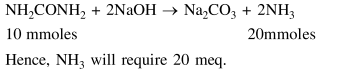# Solve this following

Question:

The ammonia $\left(\mathrm{NH}_{3}\right)$ released on quantitative reaction of $0.6 \mathrm{~g}$ urea $\left(\mathrm{NH}_{2} \mathrm{CONH}_{2}\right)$ with sodium hydroxide $(\mathrm{NaOH})$ can be neutralized by:

1. $100 \mathrm{ml}$ of $0.1 \mathrm{~N} \mathrm{HCl}$

2. $200 \mathrm{ml}$ of $0.4 \mathrm{~N} \mathrm{HCl}$

3. $100 \mathrm{ml}$ of $0.2 \mathrm{~N} \mathrm{HCl}$

4. $200 \mathrm{ml}$ of $0.2 \mathrm{~N} \mathrm{HCl}$

Correct Option: , 3

Solution: